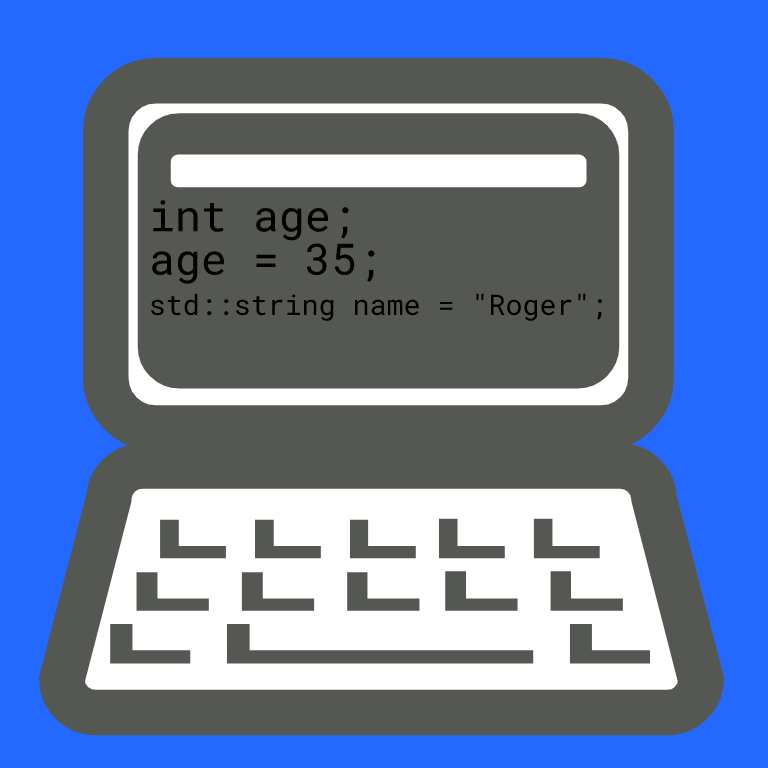# How to Create a Variable in the C++ Programming Language

Learn how

We can create variables in C++ using the following syntax:

``````TYPE varName;
``````

We declare the variable first with the data type, followed by the desired name. For example, if you want to store a person's age, you could do:

``````int age;
``````

The left-hand side is the data type: an integer. The right-hand side is the variable name.

You can assign a value to a variable like so:

``````age = 35
``````

Simply add the equal sign for the assignment operation, the value of the variable on the right-hand side. Note this is not a comparison.

You can declare your variable using other types, too. For instance, in the following examples we declare and define the values at the same time:

``````double circleArea = 3.123;

std::string name = "Roger";
``````

A `double` is a floating point type that allows for the representation of numbers that have a fractional part.

A `bool` is a boolean type that has value that is either `true` or `false`.

A `string` is a sequence of characters. The `string` type belongs to the `std` namespace.

Here is a program for you to play with

For more lessons on software development, visit NBK Tech World:BTCBCH